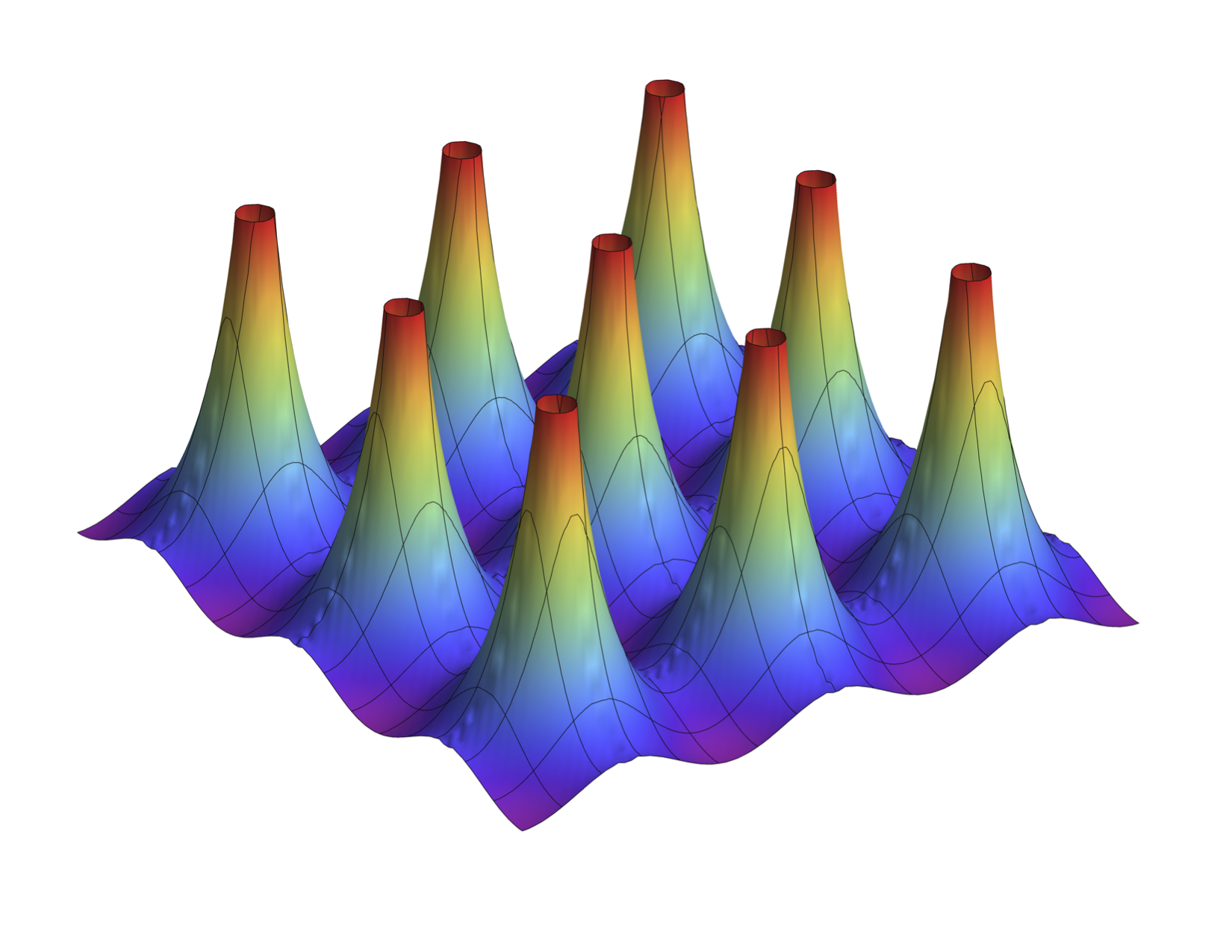# Clayton ShonkwilerShown above is the graph of the fundamental solution of the Laplacian on the flat torus (shown over 9 fundamental domains of the torus).

The fundamental solution of the Laplacian essentially inverts the Laplacian. Specifically, if a function $$f$$ is in the image of the Laplacian, then convolving $$f$$ with the fundamental solution gives the solution to the differential equation $$\Delta u = f$$ with smallest $$L^2$$ norm.

The corresponding fundamental solution on the three-dimensional torus is a key tool that we use to find an integral formula for the triple linking number. We introduced this story in our paper “Triple linking numbers, ambiguous Hopf invariants and integral formulas for three-component links”, then worked out the detail in the 3-sphere in “Generalized Gauss maps and integrals for three-component links” and in $$\mathbb{R}^3$$ in “Generalized Gauss maps and integrals for three-component links II”